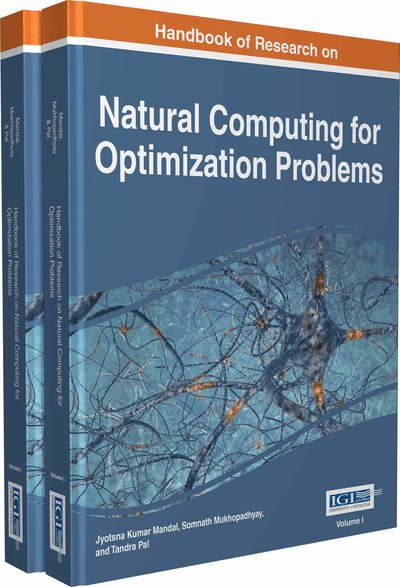# Application of Fuzzy Logic and Fuzzy Optimization Techniques in Medical Image Processing

Niladri Sekhar Datta (Future Institute of Engineering and Management, India), Himadri Sekhar Dutta (Kalyani Government Engineering College, India) and Koushik Majumder (West Bengal University of Technology, India)
DOI: 10.4018/978-1-5225-0058-2.ch033
Available
\$37.50
No Current Special Offers

## Abstract

Fuzzy logic deals with approximate rather than fixed and exact reasoning. Fuzzy variables may have a truth value that ranges in degree between 0 and 1; extended to handle the concept of partial truth where the truth value may range between completely true or completely false. This computational logic uses truth degrees as a mathematical model of the vagueness phenomenon while probability is a mathematical model of ignorance. A huge number of complex problems may be solve using Fuzzy logic specifically Fuzzy modeling and optimization method. Fuzzy modeling is the understanding of the problem and analysis of the Fuzzy information where the Fuzzy optimization solves Fuzzy model optimally using optimization techniques via membership functions. In this research article authors describe the Fuzzy rules and its application and the different types of well known problems solved by the Fuzzy optimization technique.
Chapter Preview
Top

## Introduction

Conventional optimization methods have been applied successfully for years to solve problems with a well-defined configuration known as hard systems. These optimization problems are generally well formulated by crisply specified objective functions and solved by precise mathematics. However the real world situations are often not deterministic. There exists different types of uncertainties in industrial, economic as well as social systems like imprecision, ambiguity of system, randomness of occurrence of events, data and linguistic vagueness, etc. which may grew up in many ways(Simon,1995). In general conventional optimization technique is inefficient to handle the deficiency of statistical data, incomplete knowledge expression and preference of human judgments etc. Using Fuzzy logic and Fuzzy Optimization technique different types of stochastic uncertainty may be expressed (Zimmermann, 1991). Stochastic uncertainty is related with the uncertainty of occurrences of events. These are basically descriptions of information, crisp and well defined. The Stochastic uncertainty varies in their frequency of occurrence. The systems with this type of uncertainty are called stochastic systems which can be solved by stochastic optimization technique applying probability theory. In some real scenario the decision-makers are unable to use the appropriate probability distribution particularly when the information is indistinct, relating to human behavior and language, vague system data or when the information could not be defined well due to inadequate knowledge. These types of uncertainty are classified as fuzziness which can be further classified into ambiguity or vagueness. Technically, Vagueness is the difficulty of making precise distinctions i.e. dealing with the situation where the information cannot be described clearly in linguistic term. This fuzziness is generally represented by membership function that reflects the decision-makers subjectivity and preference on the objects. Ambiguity is linked with the situation in which the choice between two or more alternatives is left undetermined. It can be further subdivided into partiality based ambiguity and possibility based ambiguity from the ways the ambiguity comes. The second one is called imprecision. Generally, the ambiguity arises from the subjective knowledge. For an example ‘the job execution time is around 25 minutes’ which is a preference-based ambiguity. That is usually expressed by the membership function. The possibility based ambiguity occurs due to incompleteness of the information. Assume the turnover of an organization is about 25 Crores. It is a possibility based ambiguity which is represented by normal intervals and it is characterized by possibility distribution that represents the possibility of occurrence of an object. The rest of the chapter is organized as follows: in the next section the state of the art are presented on this research field. In Fuzzy logic and Optimization Method section the description of Fuzzy rules with optimization method is presented. Here, in Fuzzy Optimization Method section, different types of existing Fuzzy models are highlighted. Essentially, the importance of Extremum problem, Fuzzy Mathematical Programming Problem and Fuzzy linear programming problems are described. Here, the differences between Fuzzy Symmetric and Asymmetric approaches are also presented. Then generalized approach of Fuzzy Optimization is given. After that, Fuzzy genetic algorithms with optimal solution are specified. The application of Fuzzy logic on image processing is presented thereafter. Today, in medical image analysis Fuzzy rules are very frequently used. In this section, the use of Fuzzy rules for detection of tumors and classification of normal and Diabetic eye are shown. In Fuzzy Penalty Based Approach section, the importance of penalty based method is highlighted. The Current Application of Fuzzy Logic section, the roles of Fuzzy logic and Optimization method on today’s research work is highlighted. Future Research Direction section highlights here the importance of Fuzzy rules for disease diagnostics. The Conclusion section is given before ending the chapter.

## Complete Chapter List

Search this Book:
Reset